#### Study Material and Notes of Ch 8 Motion Class 9th Science

Topics in the Chapter

• Introduction
• Distance and Displacement
• Uniform and Non-uniform motion
• Speed
• Velocity
• Accelerated and Decelerated motion
• Equations of motion
• Graphical representation of motion
• Uniform circular motion

Introduction

• Rest: A body is said to be in a state of rest when its position does not change with respect to a reference point.

• Motion: A body is said to be in a state of motion when its position change continuously with reference to a point.

→ Motion can be of different types depending upon the type of path by which the object is going through.

(i) Circulatory motion/Circular motion – In a circular path.
(ii) Linear motion – In a straight line path.
(iii) Oscillatory/Vibratory motion – To and fro path with respect to origin.

• Scalar quantity: It is the physical quantity having own magnitude but no direction. Example: distance, speed.

• Vector quantity: It is the physical quantity that requires both magnitude and direction.
Example: displacement, velocity.

Distance and Displacement

→ The actual path or length travelled by a object during its journey from its initial position to its final position is called the distance.

→ Distance is a scalar quantity which requires only magnitude but no direction to explain it.
Example: Ramesh travelled 65 km. (Distance is measured by odometer in vehicles.)

→ Displacement is a vector quantity requiring both magnitude and direction for its explanation.
Example: Ramesh travelled 65 km south-west from Clock Tower.

→ Displacement can be zero (when initial point and final point of motion are same)
Example: circular motion.Difference between Distance and Displacement

 Distance Displacement Length of actual path travelled by an object. Shortest length between initial point and far point of an object. It is scalar quantity. It is vector quantity. It remains positive, can’t be ‘0’ or negative. It can be positive (+ve), negative (-ve) or zero. Distance can be equal to displacement (in linear path). Displacement can be equal to distance or its lesser than distance.

Example 1: A body travels in a semicircular path of radius 10 m starting its motion from point ‘A’ to point ‘B’. Calculate the distance and displacement.

Solution

Total distance travelled by body, S = ?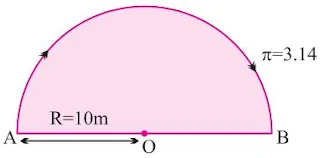Given, π = 3.14, R = 10 m
S = πR
= 3.14 × 10 m
= 31.4 m

Total displacement of body, D = ?
Given, R = 10 m
D = 2×R = 2×10 m = 20 m

Example 2: A body travels 4 km towards North then he turn to his right and travels another 4 km before coming to rest. Calculate (i) total distance travelled, (ii) total displacement.

Solution

Total distance travelled = OA + AB
= 4 km + 4 km = 8 km

Total displacement = OBUniform and Non-uniform Motions

Uniform Motion

→ When a body travels equal distance in equal interval of time, then the motion is said to be uniform motion.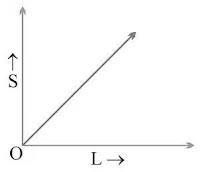Non-uniform Motion

→ In this type of motion, the body will travel unequal distances in equal intervals of time.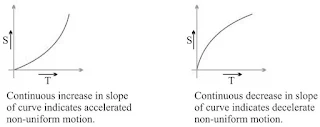Two types of non-uniform-motion

(i) Accelerated Motion: When motion of a body increases with time.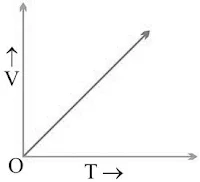(ii) De-accelerated Motion: When motion of a body decreases with time.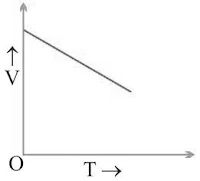Speed

→ The measurement of distance travelled by a body per unit time is called speed.

• Speed (v) = Distance Travelled/Time Taken = s/t

• SI unit = m/s (meter/second)

→ If a body is executing uniform motion, then there will be a constant speed or uniform motion.

→ If a body is travelling with non-uniform motion, then the speed will not remain uniform but have different values throughout the motion of such body.

→ For non-uniform motion, average speed will describe one single value of speed throughout the motion of the body.

• Average speed = Total distance travelled/Total time taken

Conversion Factor

• Change from km/hr to m/s = 1000m/(60×60)s = 5/18 m/s

Example: What will be the speed of body in m/s and km/hr if it travels 40 kms in 5 hrs ?

Solution

Distance (s) = 40 km
Time (t)  = 5 hrs.
Speed (in km/hr) = Total distance/Total time = 40/5 = 8 km/hr

40 km = 40 × 1000 m = 40,000 m
5 hrs = 5 × 60 × 60 sec.

Speed (in m/s) = (40 × 1000)/(5×60 ×60) = 80/36 = 2.22 m/s

Velocity

→ It is the speed of a body in given direction.
• Velocity = Displacement/Time

→ Velocity is a vector quantity. Its value changes when either its magnitude or direction changes.

→ For non-uniform motion in a given line, average velocity will be alculated in the same way as done in average speed.
• Average velocity = Total displacement/Total time

• For uniformly changing velocity, the average velocity can be calculated as follows :
Avg. Velocity (vavg) = (Initial velocity + Final velocity)/2 = (u+v)/2
where,  u = initial velocity, v = final velocity

• SI unit of velocity = ms-1

• Velocity = Displacement/Time
→ It can be positive (+ve), negative (-ve) or zero.

Example 1: During first half of a journey by a body it travel with a speed of 40 km/hr and in the next half it travels with a speed of 20 km/hr. Calculate the average speed of the whole journey.

Solution

Speed during first half (v1) = 40 km/hr
Speed during second half (v 2 )  = 20 km/hr
Average speed = (v1+v2)/2 = (40+60)/2 = 60/2 = 30

Average speed by an object (body) = 30 km/hr.

Example 2: A car travels 20 km in first hour, 40 km in second hour and 30 km in third hour. Calculate the average speed of the train.

Solution

Speed in Ist hour = 20 km/hr
Distance travelled during 1st hr = 1×20= 20 km

Speed in 2nd hour = 40 km/hr
Distance travelled during 2nd hr = 1×40= 40 km

Speed in 3rd hour = 30 km/hr
Distance travelled during 3rd hr = 1×30= 30 km

Average speed = Total distance travelled/Total time taken
= (20+40+30)/3 = 90/3 = 30 km/hr

Acceleration

→ Acceleration is seen in non-uniform motion and it can be defined as the rate of change of velocity with time.

• Acceleration (a) = Change in velocity/Time = (v-u)/t
where, v = final velocity, u = initial velocity

→ If v > u, then ‘a’ will be positive (+ve).

Retardation/Deaceleration

→ Deaceleration is seen in non-uniform motion during decrease in velocity with time. It has same definition as acceleration.

• Deaceleration (a') = Change in velocity/Time = (v-u)/t
Here, v < u, ‘a’ = negative (-ve).

Example 1: A car speed increases from 40 km/hr to 60 km/hr in 5 sec. Calculate the acceleration of car.

Solution

u = 40km/hr = (40×5)/18 = 100/9 = 11.11 m/s
v  = 60 km/hr = (60×5)/18 = 150/9 = 16.66 m/s
t = 5 sec

a = (v-u)/t = (16.66 - 11.11)/5
= 5.55/5 = 1.11 ms-2

Example 2: A car travelling with a speed of 20 km/hr comes into rest in 0.5 hrs. What will be the value of its retardation?

Solution

v = 0 km/hr
u = 20 km/hr
t = 0.5 hrs
Retardation, a’ = (v-u)/t = (0-20)/0.5
= -200/5 = -40 km hr-2

Graphical Representation of Equation

Distance-Time Graph (s/t graph)

(i) s/t graph for uniform motion: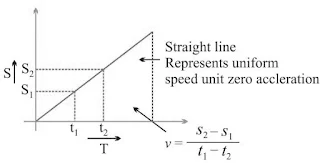(ii) s/t graph for non-uniform motion:(iii)  s/t graph for a body at rest:v = (s2 - s1)/(t2 - t1)
But, s2 - s1
∴ v = 0/(t2 - t1) or v=0

Velocity-Time Graph (v/t graph)

(i) v/t graph for uniform motion:a = (v2 - v1)/(t2 - t1)
But, v2 - v1
∴ a = 0/(t2 - t1) or a = 0

(ii) v/t graph for uniformly accelerated motion:In uniformly accelerated motion, there will be equal increase in velocity in equal interval of time throughout the motion of body.

(iii) v/t graph for non-uniformly accelerated motion: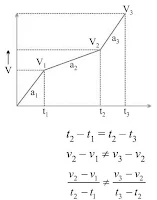a2 ≠ a1

(iv) v/t graph for uniformly decelerated motion:or, a1' = a2'

(v) v/t graph for non-uniformly decelerated motion: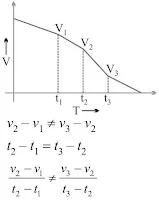a1' ≠ a2'

Note: The area enclosed between any two time intervals is ‘t2 - t1’ in v/t
graph will represent the total displacement by that body.Total distance travelled by body between t2 and t1, time intervals
= Area of ∆ABC + Area of rectangle ACDB
= ½ × (v2 – v1)×(t2 - t1) + v1× (t2 - t1)

Example: From the information given in s/t graph, which of the following body ‘A’ or ‘B’ will be more faster?Solution

vA > vB

Equation of Motion (For Uniformly Accelerated Motion)

First Equation: v = u + at

Final velocity = Initial velocity + Acceleration × Time

Graphical Derivation

Suppose a body has initial velocity ‘u’ (i.e., velocity at time t = 0 sec.) at point ‘A’ and this velocity changes to ‘v’ at point ‘B’ in ‘t’ secs. i.e., final velocity will be ‘v’.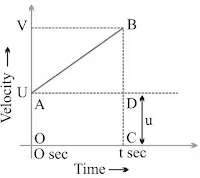For such a body there will be an acceleration.
a = Change in velocity/Change in Time
⇒ a = (OB - OA)/(OC-0) = (v-u)/(t-0)
⇒ a = (v-u)/t
⇒ v = u + at

Second Equation: s = ut + ½ at2

Distance travelled by object = Area of OABC (trapezium)
= Area of OADC (rectangle) + Area of ∆ABD
= u × t + ½ × t × (v – u)
= ut + ½ × t × at
⇒ s = ut + ½ at (∵a = (v-u)/t)

Third Equation: v2 = u2 + 2as

s = Area of trapezium OABC⇒ v2 = u2 + 2as

Example 1: A car starting from rest moves with uniform acceleration of 0.1 ms-2 for 4 mins. Find the speed and distance travelled.

Solution

u = 0 ms-1 (∵ car is at rest)
a = 0.1 ms-2
t = 4 × 60 = 240 sec.
v = ?
From, v = u + at
v = 0 + (0.1 × 240)
⇒ v = 24 ms-1

Example 2: The brakes applied to a car produces deceleration of 6 ms -2 in opposite direction to the motion. If car requires 2 sec. to stop after application of brakes, calculate distance travelled by the car during this time.

Solution

Deceleration, a = − 6 ms-2
Time, t = 2 sec.
Distance, s = ?
Final velocity, v = 0 ms-1 (∵ car comes to rest)
Now, v = u + at
Or  u = v – at
Or  u = 0 – (-6×2) = 12 ms-1
And, s = ut + ½at2
= 12 × 2 + ½ (-6 × 22)
= 24 – 12 = 12 m

Uniform Circular Motion→ If a body is moving in a circular path with uniform speed, then it is said to be executing uniform circular motion.

→ In such a motion the speed may be same throughout the motion but its velocity (which is tangential) is different at each and every point of its motion. Thus, uniform circular motion is an accelerated motion.

NCERT Solutions of Motion